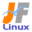Linux Kernel 2.6 Documentation: /usr/src/linux/Documentation/filesystems/tmpfs.txt

# filesystems/tmpfs.txt

tmpfs \$B%U%!%\$%k%7%9%F%`\$N@bL@(B [\$B%W%l%\$%s%F%-%9%HHG(B]
• \$B86Cx:n
• \$BK]Lu
• \$B%P!<%8%g%s(B: 2.6.12
• \$BK]LuF|;~(B: 2005/07/25
```

==================================
\$B\$3\$l\$O!"(B
linux-2.6.13-rc3/Documentation/filesystems/tmpfs.txt \$B\$NOBLu(B
\$B\$G\$9!#(B
\$BK]LuCDBN!'(B JF \$B%W%m%8%'%/%H(B < http://www.linux.or.jp/JF/ >
\$BK]LuF|(B \$B!'(B 2005/7/25
\$BK]LuA[%a%b%j\$KA4%U%!%\$%k\$rJ];}\$9\$k%U%!%\$%k%7%9%F%`\$G\$9!#(B

Everything in tmpfs is temporary in the sense that no files will be
created on your hard drive. If you unmount a tmpfs instance,
everything stored therein is lost.

tmpfs \$BFb\$N(B \$BA4\$F\$O0l;~E*\$J\$b\$N\$G!"%O!<%I%G%#%9%/>e\$K\$O%U%!%\$%kN`\$O:n@.\$5\$l\$^\$;\$s!#(B
tmpfs \$B\$r%^%&%s%H2r=|\$9\$l\$P!"\$=\$3\$KJ]B8\$5\$l\$?\$9\$Y\$F\$N\$b\$N\$O<:\$J\$o\$l\$^\$9!#(B

tmpfs puts everything into the kernel internal caches and grows and
shrinks to accommodate the files it contains and is able to swap
unneeded pages out to swap space. It has maximum size limits which can
be adjusted on the fly via 'mount -o remount ...'

tmpfs \$B\$O!"A4\$F\$r%+!<%M%k\$NFbIt%-%c%C%7%e\$KCV\$-!"4^\$^\$l\$k%U%!%\$%k\$K\$"\$o\$;\$F(B
\$BBg\$-\$/\$b>.\$5\$/\$b\$J\$j!"ITMW\$J%Z!<%8\$O%9%o%C%W%"%&%H\$5\$l\$^\$9!#(B
\$B\$=\$l\$K\$O!":GBg%5%\$%:\$N@)8B\$,\$"\$j\$^\$9\$,!"(B'mount -o remount ...' \$B\$G!"\$9\$0\$KD4@02DG=\$G\$9!#(B

If you compare it to ramfs (which was the template to create tmpfs)
you gain swapping and limit checking. Another similar thing is the RAM
disk (/dev/ram*), which simulates a fixed size hard disk in physical
RAM, where you have to create an ordinary filesystem on top. Ramdisks
cannot swap and you do not have the possibility to resize them.

(tmpfs \$B\$r:n@.\$9\$k%F%s%W%l!<%H\$G\$"\$C\$?(B) ramfs \$B\$HHf3S\$9\$k\$H!"%9%o%C%W\$H!"@)8B%A%'%C%/\$KMxE@\$,\$"\$j\$^\$9!#(B
\$B\$b\$&\$R\$H\$DJL\$NNI\$/;w\$?\$b\$N\$O!"(BRAM \$B%G%#%9%/(B( /dev/ram ) \$B\$G!"J*M}(BRAM \$BFb\$K%5%\$%:\$r(B
\$B8GDj\$7\$?%O!<%I%G%#%9%/\$r!"%7%_%e%l!<%H\$7\$^\$9\$,!":G>e0L\$K!"DL>o\$N%U%!%\$%k%7%9%F%`\$r(B
\$B:n@.\$7\$J\$1\$l\$P\$J\$j\$^\$;\$s!#(BRAM \$B%G%#%9%/\$O%9%o%C%W\$G\$-\$^\$;\$s\$7!"%5%\$%:\$NJQ99\$b\$G\$-\$^\$;\$s!#(B

Since tmpfs lives completely in the page cache and on swap, all tmpfs
pages currently in memory will show up as cached. It will not show up
as shared or something like that. Further on you can check the actual
RAM+swap use of a tmpfs instance with df(1) and du(1).

tmpfs \$B\$O!"40`z\$J7A\$G%Z!<%8%-%c%C%7%e\$H%9%o%C%W\$K>e\$K\$"\$k\$N\$G!"(B
\$B8=%a%b%jFb\$NA4\$F\$N(B tmpfs \$B%Z!<%8\$O!"%-%c%C%7%e\$5\$l\$?\$b\$N\$H\$7\$F8+\$(\$k\$G\$7\$g\$&!#(B
\$B\$=\$l\$O!"6&M-\$5\$l\$?\$b\$N(B(\$B\$^\$?\$ON`;w\$N\$b\$N(B)\$B\$H\$7\$F\$O8+\$(\$^\$;\$s!#(B
\$B\$5\$i\$K!"(Btmpfs \$B\$K\$h\$j;HMQ\$5\$l\$F\$\$\$k(B RAM+swap \$B\$O!"(Bdf(1)\$B!"(Bdu(1) \$B\$G!"D4\$Y\$k\$3\$H\$,\$G\$-\$^\$9!#(B

tmpfs has the following uses:

tmpfs \$B\$O!"0J2<\$NMQES\$r;}\$C\$F\$\$\$^\$9!'(B

1) There is always a kernel internal mount which you will not see at
all. This is used for shared anonymous mappings and SYSV shared
memory.

1) \$BA4\$/8+\$k\$3\$H\$N\$G\$-\$J\$\$%+!<%M%kFbIt%^%&%s%H\$,>o\$KB8:_\$7\$^\$9!#(B
\$B\$3\$l\$O!"F?L>\$N6&M-%^%C%T%s%0\$d!"(BSYSV \$B6&M-%a%b%j\$K;HMQ\$7\$^\$9!#(B

This mount does not depend on CONFIG_TMPFS. If CONFIG_TMPFS is not
set, the user visible part of tmpfs is not build. But the internal
mechanisms are always present.

\$B\$3\$N%^%&%s%H\$O(BCONFIG_TMPFS\$B\$K0MB8\$7\$^\$;\$s!#(B
CONFIG_TMPFS \$B\$,%;%C%H\$5\$l\$J\$\$>l9g!"(Btmpfs  \$B\$N%f!<%62D;kIt\$,AH\$_9~\$^\$l\$^\$;\$s!#(B
\$B\$7\$+\$7!"FbIt%a%+%K%:%`\$O!">o\$KB8:_\$7\$^\$9!#(B

2) glibc 2.2 and above expects tmpfs to be mounted at /dev/shm for
line to /etc/fstab should take care of this:

2) glibc 2.2 \$B0J9_\$N>l9g!"(Btmpfs \$B\$O!"(BPOSIX \$B6&M-%a%b%j(B(shm_open\$B!\$(Bshm_unlink) \$B\$N\$?\$a\$K!"(B
/dev/shm \$B\$K%^%&%s%H\$5\$l\$J\$1\$l\$P\$\$\$1\$^\$;\$s!#(B
/etc/fstab \$B\$K!"0J2<\$N9T\$rDI2C\$9\$k\$H\$-\$OCm0U\$7\$F\$/\$@\$5\$\$!'(B

tmpfs	/dev/shm	tmpfs	defaults	0 0

Remember to create the directory that you intend to mount tmpfs on
if necessary (/dev/shm is automagically created if you use devfs).

tmpfs \$B\$r%^%&%s%H\$9\$kM=Dj\$,\$"\$k>l9g!"\$=\$N\$?\$a\$N%G%#%l%/%H%j\$r:n@.\$9\$k\$3\$H\$rK:\$l\$J\$\$\$G\$/\$@\$5\$\$(B
( /dev/shm \$B\$O!"(Bdevfs \$B\$r;H\$&\$J\$i<+F0E*\$K:n@.\$5\$l\$^\$9(B)\$B!#(B

This mount is _not_ needed for SYSV shared memory. The internal
mount is used for that. (In the 2.3 kernel versions it was
necessary to mount the predecessor of tmpfs (shm fs) to use SYSV
shared memory)

\$B\$3\$N%^%&%s%H\$O!"(BSYSV \$B6&M-%a%b%j\$K\$O(B *\$BI,MW\$"\$j\$^\$;\$s(B*\$B!#(B
\$BFbIt%^%&%s%H\$,;HMQ\$5\$l\$^\$9(B( \$B%P!<%8%g%s(B2.3\$B7O%+!<%M%k\$G\$O!"(BSYSV \$B6&M-%a%b%j(B \$B\$r;H\$&\$?\$a\$K!"(B
tmpfs (shm fs) \$B\$NA0?H\$H\$7\$F!"%^%&%s%H\$r\$9\$kI,MW\$,\$"\$j\$^\$7\$?(B )\$B!#(B

3) Some people (including me) find it very convenient to mount it
e.g. on /tmp and /var/tmp and have a big swap partition. And now
loop mounts of tmpfs files do work, so mkinitrd shipped by most
distributions should succeed with a tmpfs /tmp.

3) /tmp \$B\$d(B /var/tmp\$B!"\$=\$7\$FBg\$-\$J%9%o%C%W%Q!<%F%#%7%g%s\$r;}\$C\$F\$\$\$k>l9g!"(B
\$B\$=\$l\$r%^%&%s%H\$9\$k\$N\$O\$H\$F\$bJXMx\$@\$H9M\$(\$k?M\$?\$A\$b\$\$\$^\$9!J;d\$b\$=\$&\$G\$9\$,!K!#(B
\$B\$^\$?!"8=:_!"(Btmpfs \$B%U%!%\$%k\$N(B \$B:F5"E*%^%&%s%H\$,;H\$(\$^\$9!#\$G\$9\$+\$i!"B?\$/\$N%G%#%9%H%j%S%e!<%7%g%s(B
\$B\$K4^\$^\$l\$k(B mkinitrd \$B\$O!"(Btmpfs /tmp \$B\$G\$bF0:n\$9\$k\$O\$:\$G\$9!#(B

4) And probably a lot more I do not know about :-)

4) \$B\$?\$V\$s!";d\$N\$o\$+\$i\$J\$\$\$3\$H\$,\$b\$C\$H\$?\$/\$5\$s\$"\$k\$O\$:\$G\$9(B :-)

tmpfs has three mount options for sizing:

tmpfs \$B\$K\$O%5%\$%:\$r7hDj\$9\$k\$?\$a\$K(B3\$B\$D\$N%^%&%s%H%*%W%7%g%s\$,\$"\$j\$^\$9!#(B

size:      The limit of allocated bytes for this tmpfs instance. The
default is half of your physical RAM without swap. If you
since the OOM handler will not be able to free that memory.
nr_blocks: The same as size, but in blocks of PAGE_CACHE_SIZE.
nr_inodes: The maximum number of inodes for this instance. The default
is half of the number of your physical RAM pages, or (on a
a machine with highmem) the number of lowmem RAM pages,
whichever is the lower.

size:	     \$B\$3\$N(B tmpfs \$B\$KBP\$7\$F3dEv\$F\$k%P%\$%H?t\$N>e8B!#(B
\$B%G%U%)%k%H\$O!"%9%o%C%W\$r=|\$/J*M}%a%b%j\$NH>J,!#(Btmpfs \$B%\$%s%9%?%s%9\$r8B3&\$rD6\$(\$?(B
\$BBg\$-\$5\$K\$9\$k\$H!"(BOOM \$B%O%s%I%i\$,%a%b%j\$r3+J|\$G\$-\$:%^%7%s\$,%G%C%I%m%C%/\$9\$k\$+\$b\$7\$l\$^\$;\$s!#(B
nr_brocks: size \$B\$HF1\$8\$G\$9\$,!"(BPAGE_CACHE_SIZE \$B\$N%V%m%C%/?t\$r;XDj\$7\$^\$9!#(B
nr_inodes: \$B\$3\$N%\$%s%9%?%s%9\$N(B inode \$B:GBg?t!#%G%U%)%k%H\$O!"J*M}(B RAM \$B%Z!<%8?t\$NH>J,!"\$^\$?\$O!"(B
(highmem \$B\$,\$"\$k%^%7%s\$G(B) lowmem RAM \$B%Z!<%8?t(B \$B\$N\$I\$A\$i\$+>.\$5\$\$J}!#(B

These parameters accept a suffix k, m or g for kilo, mega and giga and
can be changed on remount.  The size parameter also accepts a suffix %
to limit this tmpfs instance to that percentage of your physical RAM:
the default, when neither size nor nr_blocks is specified, is size=50%

\$B\$3\$l\$i%Q%i%a!<%?\$O!"(Bk\$B!"(Bm\$B!"(Bg(\$B\$=\$l\$>\$l!"%-%m!"%a%,!"%.%,(B) \$B\$r%5%U%#%C%/%9\$G\$-!"(B
\$B:F%^%&%s%H;~\$KJQ99\$b\$G\$-\$^\$9!#(Bsize \$B%Q%i%a!<%?\$O!"J*M}(BRAM\$B\$N\$KBP\$9\$k3d9g\$r(B
tmpfs \$B%\$%s%9%?%s%9\$N>e8B%5%\$%:\$K\$9\$k\$?\$a\$K!"(B% \$B\$b;H\$(\$^\$9!'(Bsize\$B!"(Bnr_brocks \$B\$N(B
\$B\$I\$A\$i\$b;XDj\$7\$J\$\$>l9g\$N%G%U%)%k%H\$O!"(Bsize=50% \$B\$G\$9!#(B

If nr_blocks=0 (or size=0), blocks will not be limited in that instance;
if nr_inodes=0, inodes will not be limited.  It is generally unwise to
mount with such options, since it allows any user with write access to
use up all the memory on the machine; but enhances the scalability of
that instance in a system with many cpus making intensive use of it.

nr_blocks=0 ( \$B\$^\$?\$O!"(Bsize=0 ) \$B\$N>l9g!"\$=\$N%\$%s%9%?%s%9\$G\$O!"(Bblock \$B?t\$OL5@)8B\$K\$J\$j\$^\$9!((B
nr_inode=0 \$B\$N>l9g!"(Binode \$B?t\$,L5@)8B\$G\$9!#0lHLE*\$K\$3\$l\$i%*%W%7%g%s\$r;H\$C\$F%^%&%s%H\$9\$k\$3\$H\$O!"(B
\$B6r\$+\$J\$3\$H\$G\$9!#=q9~\$_8"\$r;}\$D%f!<%6\$,!"%^%7%s>e\$N%a%b%j\$r;H\$\$2L\$?\$7\$F\$7\$^\$&\$+\$i\$G\$9!#(B
\$B\$7\$+\$7!"\$=\$l\$r=8CfE*\$K;HMQ\$9\$k!"\$?\$/\$5\$s\$N(B CPU \$B\$r;}\$D%7%9%F%`\$G!"\$=\$N%\$%s%9%?%s%9\$N(B
\$B%9%1!<%i%S%j%F%#\$rA}\$7\$^\$9!#(B

To specify the initial root directory you can use the following mount
options:

\$B=i4|%k!<%H%G%#%l%/%H%j\$r;XDj\$9\$k\$?\$a!"e\$G!"(Bchmod(1), chown(1), chgrp(1)
\$B\$K\$h\$C\$FJQ99\$G\$-\$^\$9!#(B

So 'mount -t tmpfs -o size=10G,nr_inodes=10k,mode=700 tmpfs /mytmpfs'
will give you tmpfs instance on /mytmpfs which can allocate 10GB
RAM/SWAP in 10240 inodes and it is only accessible by root.

\$B\$h\$C\$F!"(B 'mount -t tmpfs -o size=10G,nr_inodes=10k,mode=700 tmpfs /mytmpfs'
\$B\$G\$O!"(B /mytmpfs \$B>e\$N(B tmpfs \$B%\$%s%9%?%s%9\$G!"(B10240\$B0J2<\$N(B inode \$B\$G(B RAM/SWAP \$B\$K(B
10GB \$B3dEv\$F\$G\$-!"\$^\$?!"(Broot \$B\$@\$1\$,%"%/%;%9\$G\$-\$^\$9!#(B

Author:
Christoph Rohland <cr at sap dot com>, 1.12.01
Updated:
Hugh Dickins <hugh at veritas dot com>, 13 March 2005
```